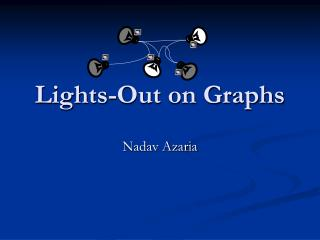# Lights-Out on Graphs - PowerPoint PPT PresentationDownload PresentationLights-Out on Graphs

Lights-Out on GraphsDownload Presentation## Lights-Out on Graphs

- - - - - - - - - - - - - - - - - - - - - - - - - - - E N D - - - - - - - - - - - - - - - - - - - - - - - - - - -
##### Presentation Transcript

1. Lights-Out on Graphs Nadav Azaria

2. What is “Lights-Out” ? • “Lights-Out” is a hand-held electronic game by Tiger electronics. It is played on a 5‰5keypad of lightable buttons. • Other versions of the game exist.

3. On Start: • Some random buttons are lit. Object: • To turn all the lights out on the keypad. The difficulty is that each time you press a lit or an unlit button, it not only changes that button, but also all adjacent buttons!

4. Lets Play!

5. From ToysRus to BGU The game gave inspiration to several researches.

6. Lights-Out on Arbitrary Graphs Let G=(V,E) be a given graph. Suppose that at each vertex there is a light bulb and a switch. Toggling the switch at a vertex, we flip the light at this vertex and all its neighbors - those that were off are turned on and vice versa. A configuration of the system is a point of {0,1}V, where a 0 coordinate indicates that the light at the corresponding vertex is off, while a 1 means that it is on.

7. Did you notice that… While solving the game: • There is no point pressing the same button more than once. • The order in which you press the buttons has no effect on the final configuration. Thus: A solution may be identified with a subset of V.

8. Back to the previews example

9. Question • Given two configurations, decide whether it is possible to pass from one to the other by some sequence of switch toggles.

10. Answer • Let M(G) be the neighborhood matrix of G. • If C is some configuration and we press some vertex v, the resulting configuration is C+M(G)v, where M(G)v is the row of M(G) corresponding to v.

11. Press (0,1,0,0) (0,1,1,1) (0,0,1,1) (0,0,1,1) (1,1,0,0) (1,1,1,1) Press

12. Meaning…. We can pass from C1 to C2 if and only if there exists an xÎ{0,1}V such that C1 + M(G)x= C2 Or, equivalently: M(G)x= C2 - C1

13. Conclusions We can now always assume starting with the all-off configuration and only ask which configurations can be reached. AND All configurations can be reached M(G) is non- singular over Z2

14. The End ?! We are interested in: We are interested in: Which graphs have the property that one can pass from any configuration to any other? Which graphs have the property that one can pass from any configuration to any other?

15. Oh Yes, and find algorithms for evaluating light-deficiency for specific graph types. Naturally they need to perform better then O(n2.376).

16. Definitions A 0-combination is a non-zero vector in Ker(M(G)). For example, in the graph , (1,1) is a 0- combination. A graph is light-transitive if each configuration can be reached. v1 v2

17. Thelight-deficiencyd(G) of G is the dimension of the kernel of M(G). Thus, there exist 2|V|- d(G) reachable configurations.

18. Universal Configurations • A universal configuration is a non-trivial configuration which is reachable for each graph. • TheoremThe all-on configuration is the only universal configuration.

19. Invariant Graphs (soon) A rooted union of two rooted graphs:

20. Invariant Graphs An invariant graphI satisfy d(GÇ I)=d(G), for any rooted graph G. Ç - rooted union. r r

21. Q. When does a light-transitive rooted graph is invariant? A. A light-transitive rooted graph is invariant •The configuration which all lights if off except for the root, must be lit using the root itself.

22. Example: r Actually, any light-transitive cycle with an arbitrary vertex as the root is invariant.

23. S1 S2 S3 r r r

24. Meaning… G’ G The number of unreachable configuration in G stays the same .

25. Introducing S5… r A 0-combination

26. Now, what do I know about G’? G’ The same 0-combination Thus, G’ is not light-transitive!

27. r r Proposition:Let G = (V, E, r) be any rooted graph. Then G The number of 0-combination now is exactly half

28. The Tree’s Theorem • Any tree T with at least three vertices a rooted union of some rooted tree T’ and one of the four rooted trees S1,S2,S3,S5. Proof: S2 S3 S1 S5

29. The Algorithm The Goal: • Given a tree, evaluate its light-deficiency. The Means: • Assign a status to each vertex. The status of a vertex determined by the status of its sons. A status is pair consist of an integer and one of the following: • Null • Leaf • Father

30. Status? Add 1 to the final result (Null, 0) (Father, 1) (Leaf, 0)

31. Ha,ha My status is (Null, 9) What is my status? The leaves … The fathers… The nulls… 1 2 0 3 0 0 0 1 Remember : 3 9

32. See it in action… (Null, 2) (Father, 1) (Null, 0) (Father, 0) (Father, 0) (Leaf, 0) (Leaf, 0) (Null,1) (Leaf, 0) (Leaf, 0) (Father, 0) (Father, 0) (Leaf, 0) (Leaf, 0)